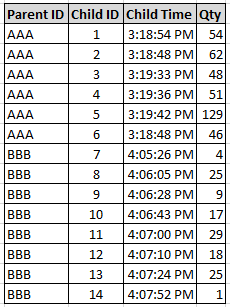# App Development

Announcements
Read about the latest Qlik Community enhancements on the Community News blog!
cancel
Showing results for
Did you mean:Contributor III

## Calculating time as a percentage per dimension

Hi, I've spent a couple of days on this problem now, and can't find a solution.

So I have two tables.

My parent order table:and my child order tableBasically, what I am trying to do here is calculate the child time as a percent, aggregated by Parent ID. To do this, I calculate the lifetime of the order in seconds (Max ChildTime - Min ChildTime) for each Parent ID, then calculate the time into the life of the order of the child in seconds (ChildTime - MinTime) for each Parent ID, then divide the time of the child by the life time of the order, that gives me the figure I want (mathematically at least).

In QlikSense I have the below:``Child Time in Second = Num(ChildTime) *24*60*60``
``First Fill in Second = Num(Aggr(Min(ChildTime),[Ord ID])) *24*60*60``
``Last Fill in Second = Num(Aggr(Max(ChildTime),[Ord ID])) *24*60*60``

But for SOME REASON, when  I try to find my Child Time - First Fill  with this, only a single value shows for the dimension, as shown on the table above

``=(Num(ChildTime) *24*60*60) - (Num(Aggr(Min(ChildTime),[Ord ID])) *24*60*60)``

Does anyone have any idea why this is happening? Or how I can achieve what I described.

Thanks!!!

0 RepliesTags
Community Browser